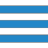# SQL SELECT with DISTINCT on multiple columns## Description

Multiple fields may also be added with DISTINCT clause. DISTINCT will eliminate those rows where all the selected fields are identical.

Here is an simple query on some selected columns in orders table where agent_code='A002'

## Sample table : orders

SQL Code:

``````SELECT agent_code, ord_amount, cust_code, ord_num
FROM orders WHERE agent_code='A002';
``````

Output:The above picture shows the same agent_code, ord_amount and cust_code appears more than once in the orders table.

## Example : select with distinct on two columns

To get the identical rows (based on two columns agent_code and ord_amount) once from the orders table , the following sql statement can be used :

SQL Code:

``````SELECT  distinct agent_code,ord_amount
FROM orders
WHERE agent_code='A002';
``````

Output:## Pictorial presentation of above query :## Example : select with distinct on three columns

To get the identical rows (based on three columns agent_code, ord_amount and cust_code) once from the 'orders' table , the following sql statement can be used :

SQL Code:

`````` SELECT  distinct agent_code, ord_amount,cust_code
FROM orders
WHERE agent_code='A002';
``````

Output:## Pictorial presentation of above query :## Example : select with distinct on all columns of the first query

To get the identical rows (on four columns agent_code, ord_amount, cust_code and ord_num) once from the 'orders' table , the following sql statement can be used :

``````select distinct agent_code,ord_amount,cust_code,ord_num  from orders
WHERE agent_code='A002';
``````

Output:In the above output, all rows whose agent_code is 'A002' have returned because there is no identical rows on agent_code, ord_amount, cust_code and ord_num. See the following presentation :

## Pictorial presentation of above query :## Select with distinct on multiple columns and order by clause

You can use an order by clause in select statement with distinct on multiple columns. Here is an example :

``````  SELECT  distinct agent_code,ord_amount
FROM orders WHERE agent_code='A002' order by ord_amount;``````

Output:## Count() function and select with distinct on multiple columns

You can use count() function in a select statement with distinct on multiple columns to count the distinct rows. Here is an example :

`````` select count(*) from (
SELECT  distinct agent_code, ord_amount,cust_code
FROM orders WHERE agent_code='A002');
``````

Output:Outputs of the said SQL statement shown here is taken by using Oracle Database 10g Express Edition.

﻿